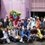# Simple Permutation Problem

Hello everyone, I have a little problem in permutation. Here's the problem: From the sets of numbers: 2,3,4,5,6,7,9, we want to create a number with a criteria:

1. The number is consist at least three digits

2. The number is less than 400

3. The number must be even

4. All the digits are different, i.e you can create 234 but cannot create 244

By using the methods of counting, I got 30 as an answer. But, is it true? And, if I want to use permutation formula to solve this problem, how can I apply it to get the same answer with the methods of counting? ThanksNote by Leonardo Chandra
7 years ago

This discussion board is a place to discuss our Daily Challenges and the math and science related to those challenges. Explanations are more than just a solution — they should explain the steps and thinking strategies that you used to obtain the solution. Comments should further the discussion of math and science.

When posting on Brilliant:

• Use the emojis to react to an explanation, whether you're congratulating a job well done , or just really confused .
• Ask specific questions about the challenge or the steps in somebody's explanation. Well-posed questions can add a lot to the discussion, but posting "I don't understand!" doesn't help anyone.
• Try to contribute something new to the discussion, whether it is an extension, generalization or other idea related to the challenge.

MarkdownAppears as
*italics* or _italics_ italics
**bold** or __bold__ bold
- bulleted- list
• bulleted
• list
1. numbered2. list
1. numbered
2. list
Note: you must add a full line of space before and after lists for them to show up correctly
paragraph 1paragraph 2

paragraph 1

paragraph 2

[example link](https://brilliant.org)example link
> This is a quote
This is a quote
    # I indented these lines
# 4 spaces, and now they show
# up as a code block.

print "hello world"
# I indented these lines
# 4 spaces, and now they show
# up as a code block.

print "hello world"
MathAppears as
Remember to wrap math in $$ ... $$ or $ ... $ to ensure proper formatting.
2 \times 3 $2 \times 3$
2^{34} $2^{34}$
a_{i-1} $a_{i-1}$
\frac{2}{3} $\frac{2}{3}$
\sqrt{2} $\sqrt{2}$
\sum_{i=1}^3 $\sum_{i=1}^3$
\sin \theta $\sin \theta$
\boxed{123} $\boxed{123}$

Sort by:

The answer is actually 25, I believe. Clearly, the hundreds place must contain a $2$ or a $3$. If it contains a $2$, we can put either a $4$ or a $6$ in the units place and then any of the five remaining numbers in the tens place. This gives $10$ possibilities. If the hundreds place contains a $3$, then we can fill in the units place with a $2$, a $4$, or a $6$ and the tens place with any of the five remaining numbers. This gives $15$ possibilities. $10 + 15 = \fbox{25}$. I think you counted the numbers which begin and end in $2$, which are not allowed but would give an answer of $30$ if they were included.

- 7 years ago

Yeah I think your's is right.

- 7 years ago

Considering the conditions, you may form one-digit numbers, two-digit number, or three-digit numbers. 1) For one-digit numbers, you may choose from 2, 4, or 6. Which means there are 3 possible numbers. 2) For two-digit numbers, you may only choose from either 2,4, or 6 to be your units digit. Which means you have 3 choices. For the tens digit, since a digit has been chosen as your ones digit, there will only be 6 choices left. Which means there will be 63 or 18 two digit numbers. 3) For the three-digit numbers. You only have two numbers as your choice for the hundreds digit since the numbers have to be less than 400. These are 2 or 3. However, choosing either will affect the number of possible even digits for the ones. So, it will be broken down into two cases: first, a number that starts with 2 and second, a number that starts with 3. For the first case since the first digit is 2, there will be two choices for the ones digit (4 or 6). Therefore, there will only be five choices for the tens. Hence, there will be 152 or 10 numbers. For the second case since the first digit is 3, there will be three choices for the ones digit (2, 4, or 6), and since two digits have been chosen for the hundreds (3) and ones (2,4, or 6), there will only be five choices for the tens. Therefor there will be 15*3 or 15 numbers. 4) All in all there will be 3+18+10+15 or 46 numbers. If you list down the numbers they are: 2,4, and 6 for a single-digit number. 24, 26, 32, 34, 36, 42, 46, 52, 54, 56, 62, 64, 72, 74, 76, 92, 94, and 96 for the two-digit numbers. 234, 236, 246, 254, 256, 264, 274, 276, 294, and 296 that starts with 2. 324, 326, 342, 346, 352, 354, 356, 362, 364, 372, 374, 376, 392, 394, and 396 that starts with three.

- 5 years, 10 months ago

Sorry, some typo errors. 63 is supposed to be 63, 152 is supposed to be 152, and 153 should be 153. Thanks!

- 5 years, 10 months ago

Ooops! Again! 63 is 6 times 3, 152 is 1 times 5 times 2. and 15*3 is 1 times 5 times 3. There is a problem with the symbol for asterisk. I don't know why. Thanks again.

- 5 years, 10 months ago